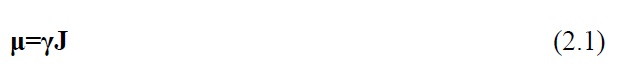## MRI Signal

#### NMR Phenomenon

The physical phenomenon behind MRI is nuclear magnetic resonance (NMR), which was first discovered in the 1940s. An atomic nucleus with an odd number of protons possesses an angular momentum J called spin, which generates a tiny magnetic moment μ. The magnetic moment is directly proportional to the angular moment asHere γ is a constant called the gyromagnetic ratio. The proton in hydrogen (1H) is particularly interesting because it is abundant in water and other molecules in the human body. At room temperature and without a strong external magnetic field, the spins are randomly oriented and their magnetic moments have a vector sum of zero.Fig. 2.1. The protons alignment. There is always a fact that the protons align with the external field is a little bit more than that of the protons align against the field

When the spins are placed in a strong external magnetic field, they align themselves with, as shown in Fig. 2.1. In fact, in the so-called thermal equilibrium state, proton spins are divided among two populations, one population (n+) oriented parallel and the other (n-) oriented antiparallel to. (In general, at thermal equilibrium, spins populate their quantized energy states according to a Boltzmann distribution; for spin-1/2 species such as 1H, there are two such states corresponding to oppositely oriented angular momenta.) The n+ spin population is at a relatively lower energy state and thus has a slightly larger number of spins than the n- spin population. This results in a net magnetization vector M0, which is the vector sum of all the spin angular momenta and is aligned in the direction of, known as the z direction or longitudinal direction. The magnitude ofcan be calculated asHere h is Planck’s constant, K is the Boltzmann’s constant and T is the absolute temperature. Eq. (2.2) suggests that the net magnetization that can be measured in MRI is proportional to the magnetic field. When spins are perturbed away from the axis of the applied B0 field, they precess around the direction ofat a frequency that is proportional to the strength of, as given by the Larmor equation## Signal Excitation

In order to generate MR signal that can be measured by a detector, the net magnetizationneeds to be tipped towards the direction perpendicular to, which is known as the x-y plane or transverse plane. This process is known as “excitation” and is achieved by applying a radiofrequency (RF) pulse that creates a magnetic field () perpendicular toand rotating at the Larmor frequency. The RF pulse causes M0 to move away from the z direction into the transverse plane until the pulse is switched off. The nutation angle through which M0 moves, or the “flip angle” of the pulse, is given byHere B1 is the strength of the RF magnetic field and T is the duration of the pulse. Following the excitation, the excess z-population M0 is at least partially converted into a transverse magnetization component (??? ), as shown in Fig. 2.2, and the ensemble of spins retain their
relative alignment, or phase coherence. The precession of ??? generates an oscillating magnetic field, and the changing magnetic flux associated with this field can then induce a voltage in a suitably configured receive coil. This voltage constitutes the MR signal, which can subsequently be demodulated or manipulated otherwise as desired.Fig. 2.2. Following the excitation, the excess z-population is at least partially converted into a transverse magnetization component (??? ), and the ensemble of spins retain their relative alignment, or phase coherence.

## Relaxation

After the RF pulse is switched off, the precession spins gradually lose their coherence and return to the z-directed equilibrium state, in processes known collectively as relaxation. The loss of spin coherence results from differences in local field strength and precession frequency, or else from other interactions between spins and their environment, and it is characterized by a time constant. The return to longitudinal equilibrium is associated with loss of the energy the spins absorbed from the RF pulse, and is characterized by a time constant. These two relaxation mechanisms, together with the behavior of the magnetization vector when exposed to an external magnetic field, can be described by the Bloch equation :Here M = (Mx, My, Mz), and i, j, and k are unit vectors along x, y, and z directions respectively. The cross-product term describes the precession behavior and the relaxation terms describe the exponential behavior of transverse dephasing and longitudinal recovery. For a flip angle of 90o, the solution to Eq. (2.5) is given by## Slice Selection

An RF pulse is usually designed at the resonance condition, with a bandwidth centered at the Larmor frequency. Thus, without the presence of gradients, the RF pulse excites all the spins in
the object being imaged since they are all rotating at the same frequency. In order to excite a slice in 2D MR imaging, a slice-selective gradient () needs to be first imposed along an axis perpendicular to the imaging slice to be excited, so that the Larmor frequency of the spins varies along that axis. Besides, a specially tailored RF pulse needs to be applied simultaneously with the gradient, with its frequency bandwidth matching the range of frequencies contained in the desired slice. This slice-selective excitation ensures that only spins within a slice or slab of interest can be excited, while leaving other spins unperturbed. Accordingly, the relationship between slice thickness and RF bandwidth or gradient strength can be given byhttps://search.proquest.com/openview/eec314ed838aba2c6d50ce7f75b7e2c2/1.pdf?pq-origsite=gscholar&cbl=18750&diss=y